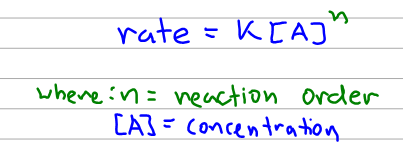# Problem: At 700 K acetaldehyde decomposes in the gas phase to methane and carbon monoxide. The reaction is: CH3CHO(g) → CH4(g) + CO(g). A sample of CH3CHO is heated to 700 K and the pressure is measured as 0.31 atm before any reaction takes place. The kinetics of the reaction are then followed by measurements of total pressure and these data are obtained:t (s)010003000  7000  PTotal (atm)0.31  0.35  0.400.46Find the rate law.

###### FREE Expert Solution

The general formula for the rate law of a reaction with a single reactant is shown below:Now the complication here is that we are given pressure. We can, however, relate the concentration and pressure using the ideal gas law.###### Problem Details
At 700 K acetaldehyde decomposes in the gas phase to methane and carbon monoxide. The reaction is: CH3CHO(g) → CH4(g) + CO(g). A sample of CH3CHO is heated to 700 K and the pressure is measured as 0.31 atm before any reaction takes place. The kinetics of the reaction are then followed by measurements of total pressure and these data are obtained:
 t (s) 0 1000 3000 7000 PTotal (atm) 0.31 0.35 0.4 0.46

Find the rate law.